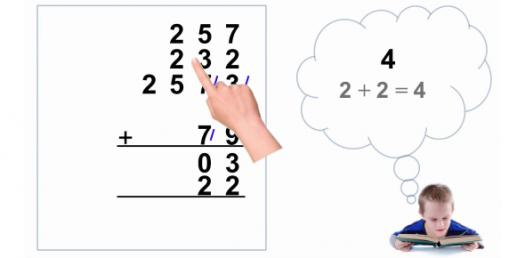48 Questions | Total Attempts: 118SettingsThis trivia is used to test the concepts of math, mainly addition. Math is a basic life skill as we need it in our everyday life, from grocery shopping to paying bills. Because of this, it is important that you grasp all the concepts in mathematics and how to solve some common problems. Take our trivia and improve your skills.

• 1.
9+9=
• 2.
0+8=
• 3.
7+7=
• 4.
8+9=
• 5.
5+9=
• 6.
8+6=
• 7.
7+5=
• 8.
8+5=
• 9.
8+7=
• 10.
6+7=
• 11.
9+9=
• 12.
7+9=
• 13.
8+7=
• 14.
9+5=
• 15.
5+8=
• 16.
6+6=
• 17.
6+9=
• 18.
9+9=
• 19.
8+7=
• 20.
6+6=
• 21.
7+8=
• 22.
5+7=
• 23.
0+6=
• 24.
6+8=
• 25.
9+9=
Related TopicsBack to top